6. Continuous and Discontinuous Differentials and Derivatives of Judgements of Dialectical Logic

L. G. Kreidik (translation from Russian T. S. Kortneva and G. P. Shpenkov)

6.1. Material and ideal points

Adequate descriptions of objective-subjective processes are based on the concept of material and ideal points:

An ideal point xi is the limit of the sequences of enclosed volumes of material points, when the largest cross-section of the volumes tends to zero. The position of an ideal point is defined by its mathematical coordinates. In particular, if a point is on the x-axis then its coordinate and the point itself will be denoted by the same symbol xc.

A material point xm is any object with a very small volume, the size of which must not be neglected. A material point position and size will be defined by an arbitrary ideal point. In particular, if the material point is on the .x-axis, its position and size will have the form of the interval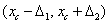, where xc is some ideal point of the material point xm. Thus, in the case of the linear material point, we have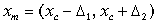. (1.106)

Material points will be denoted for short by the same symbol xc as ideal points.

Mutual additive parameters of the points and relations within material points will be expressed by additive differentials. There are four main types of the additive differentials: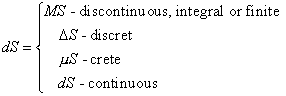. (1.107)

The first differential, MS, describes finite variations, the second, D S, describes minor variations, the third, m S, describes minute variations, the fourth dS describes variable variations, assuming any infinitesimal values, including zero at the corresponding point of a judgement. Of course, such partition of differentials on the four types has relative character.

Additive variations (differentials) will be supplemented by inverse additive variations-differentials at an arbitrary point t: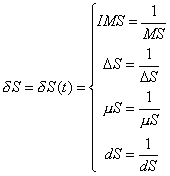(1.108)

and at a definite point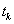: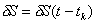, (1.109)

whereis some characteristic ideal point of the material point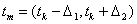.

6.3. A dditive derivatives

The generalized additive derivative is defined by the expression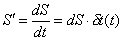, (1.110)

where dS and dt are differentials of any types and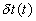is the inverse differential.

If the differentials are continuous, the limit of the relation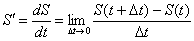(1.111)

defines a continuous derivative. Differentials of the same type form average derivatives and differentials of different types define derivatives of different degrees of discreteness: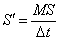,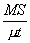,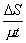,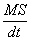,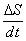,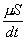, (1.112)

where dt is some small increment of the argument t. These derivatives will be called discontinuous, discrete, indiscrete, etc.

Material and ideal points, at which a judgement has a continuous derivative, are points of continuous change or continuous transition. Material points with discontinuous derivatives define points of discontinuous change or transition. Discontinuous derivatives will be expressed in the form: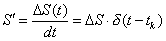, (1.113)

where dt is the change in the t variable within the material point of the discontinuous transition of the judgement and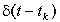is the inverse differential. Contraction of the material point to an ideal one results in an ideal discontinuous derivative.

6.4. Derivatives and character of variation of judgements

As oppositi-judgements, derivatives allow us to express judgements about the behavior of the judgement, which visually can be expressed by a plot of the oppositus and its derivative.

We are interested primarily in the points, at which judgements experience breaks and sudden change, expressing continuous-discontinuous transitions in nature. Let at the material point xm a judgement-oppositus undergoes discontinuous transition on the qualitative side and continuous transition on the quantitative side. Graphically it is expressed by a break (Fig. 1.8). A judgement with a break is described by the logical structure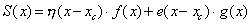, (1.114)

where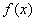and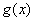are oppositi that determine the behavior of the judgementbefore and after the transition;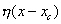and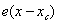- are unit step-judgements: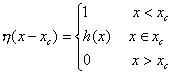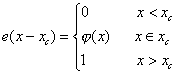, (1.115)

where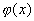and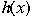are functions at the point.

The step-judgements describe sharp transitions from 0 to 1 and from 1 to 0 within the material point.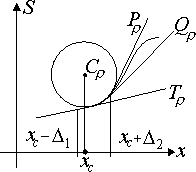Fig. 1.8. The oppositus and its derivatives at the domain of break.

These transitions will be called discontinuous, discrete, or indiscrete, depending on the degree of their steepness. These are real contradictory transitions, discreteness of which is realized through continuity.

If a material point contracts to an ideal point, step-judgements become ideal step-judgements. The behavior of function (1.114) will be expressed by the simplified model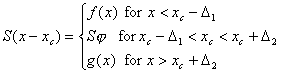(1.116)

where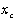is a limiting point of a material point of transition;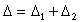is the range of the transition;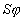are values of the judgement within the range of the transition and laying on the arc of the circle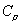with the radius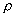, symbolizing the material point of the graph of the judgement (Fig. 1.8).

The values of the judgement on the left and on the right from the break point will be assumed to be equal to the value of the judgement at that very point to within variations of the judgement in limits of this point: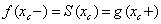, (1.117)

The values of the derivatives on the left and on the right of xc are not equal: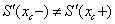. (1.118)

When the radiusis small, the judgement S has, at the material point xm

a) the left derivative determining the slope of the tangent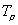: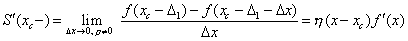; (1.119)

b) the right derivative determining the slope of the tangent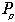: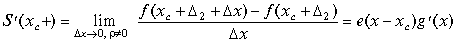(1.120)

a set of intermediate derivatives along the arc S, whose values fill continuously the interval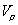: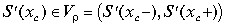(1.121)

and determine the set of slopes of the intermediate tangents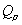.

At the material point of break, the derivative can be expressed by the functional structure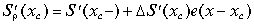,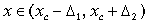, (1.122)

where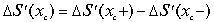is a jump of the derivative.

Over the whole range of the judgement change we have: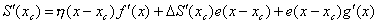, (1.123)

where the first and third terms determine the change of the derivative before and after the jump.

If we assume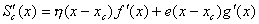, (1.124)

then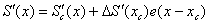. (1.125)

Here the first term is a continuous component of the derivative outside the material point. Within the material point, the second term determines the discrete component of the derivative. Thus, the derivative defined by (1.125) is a continuous-discontinuous derivative.

If the derivative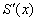does not have a break at the material point, i.e. its left and right erivatives are equal: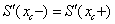, then at the material point the second derivative becomes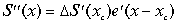. (1.126)

6.5. A generalized in verse differential

Since at the material point xm the average derivative of a unit step-judgement is equal to the inverse differential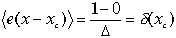, where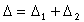(1.127)

(see Fig. 1.8), it seems reasonable to introduce a generalized inverse differential, defining it as the inverse differential-function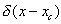, equal to the derivative of the unit step-judgement at the material point xm :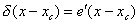(1.128)

Thus, we can speak about two inverse differentials: a differential-average derivative and a differential-instantaneous derivative at the material point xm. For simplicity, in what follows inverse differentials of both types will be called identically as inverse differentials. When the material point xm contracts to an ideal point xc , the inverse differential-function becomes a limiting inverse ideal differential equal to the d -function.

In terms of the inverse differential, the second derivative of a judgement at the material point will be expressed in the form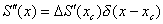. (1.129)

The plot of the inverse differential-function shaped as a compact pulse determines completely all changes of the nth derivative at xm if any breaks of the derivatives are lacking: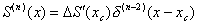(1.130)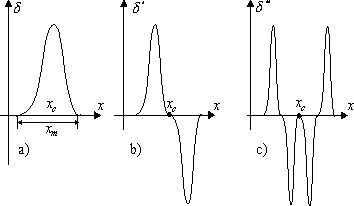Fig. 1.9. The inverse differential and its two first derivatives.

Figure 1.9 shows plots of the inverse differential-function and its two derivatives, first and second. They show the behavior of the derivatives at the points of a pure break, described by the present simplified model of the break, to within a constant factor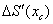.

6.6. A total derivative of a judgement

For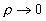, the family of the oppositi S with their derivatives becomes an oppositus with an ideal absolutely sharp break (Fig. 1.10).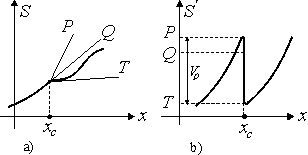Fig. 1.10. The function S and its derivative S' in the region of an ideal break.

At the point of an ideal mathematical break, the opposite has:

a) the left derivative determining the slope of the tangent P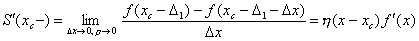; (1.131)

b) the right derivative determining the slope of the tangent T: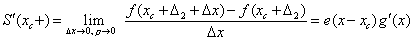(1.132)

c) a set of intermediate derivatives , whose values fill continuously the interval: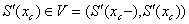(1.133)

and determine a set of slopes of intermediate tangents Q .

If at the material point the conditions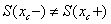and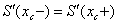, (1.134)

are satisfied, the judgement undergoes discrete transition with a discrete derivative at the transition point: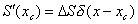, where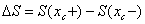. (1.135)

This transition will be called a jump at the material point (Fig. 1.11). In the transition, there occurs informal quantitative discontinuity and qualitative continuity.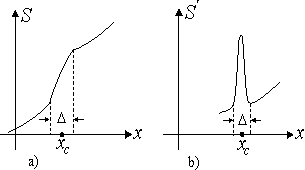Fig. 1.11. The function S and its derivative S' in the region of a jump.

The total derivative of a judgement with a jump has the form: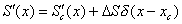, (1.136)

where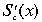is a continuous component of the derivative;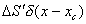is a discontinuous component of its.

Accordingly, the n-th derivative of the jump has the form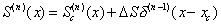. (1.137)

A complete discrete transition (a jump with a break, Fig.1.12) is characterized by the condition: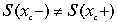and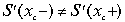. (1.138)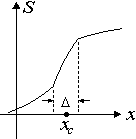Fig. 1.12. Function S in the region of a jump with a break

In the transition the first derivative contains one continuous term and two discrete ones, determining the qualitative and quantitative discreteness: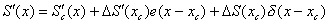. (1.139)

Differentiation of the first derivative gives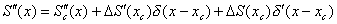. (1.140)

and so on.

There are also the following types of discreteness of the judgement S:

a) a pulse (a double jump) (Fig. 1.13a)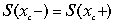,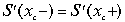Ķ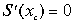, 1.141)

b) a pulse with a break (Fig. 1.13b),and, (1.142

c) a pulse with a jump (Fig. 1.13c)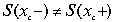,and, (1.143)

d) a pulse with a break and a jump (Fig. 1.13d),and. (1.144)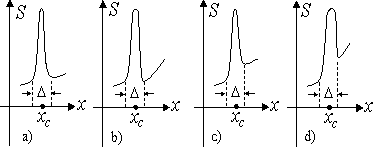Fig. 1.13. Possible types of discreteness of a judgement S.

As the cross-section of a material point increases, discreteness spreads, becoming continuity.

Theoretical Dialectical Journal: Physics-Mathematics-Logic-Philosophy, N.1, site http://www.tedial.narod.ru/